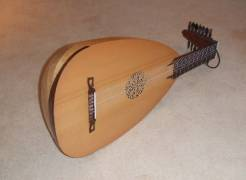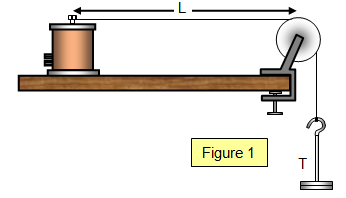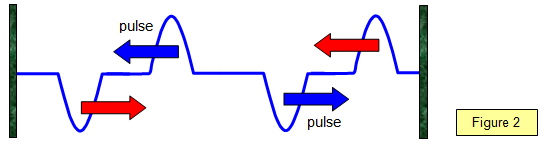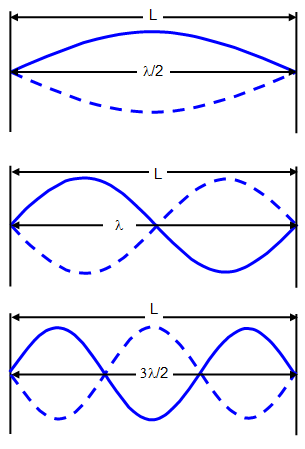Vibrating stringsIf a string stretched between two points is plucked it vibrates, and a wave travels along the string. Since the vibrations are from side to side the wave is transverse. The velocity of the wave along the string can be found as follows.

Velocity of waves along a stretched string

Assume that the velocity of the wave v depends upon
(a) the tension in the string (T),
(b) the mass of the string (M) and
(c) the length of the string (L) (see Figure 1).

Therefore: v = kTxMyLz

Solving this gives x = ˝ , z = ˝ , y = - ˝ .
The constant k can be shown to be equal to 1 in this case and we write m as the mass per unit length where m = M/L. The formula therefore becomes:

velocity of waves on a stretched string = [T/m]1/2Since velocity = frequency x wavelength

Frequency of a vibrating string = λ[T/m]1/2schoolphysics: Standing waves video

To see a video of standing waves please click on the video link.

Example problems
1. Calculate the fundamental frequency for a string 0.45 m long, of mass 0.5 gm/metre and a tension of 75 N.

f = 1/2L[T/m]1/2 = 1/0.9x75/[0.5x10-3]1/2 = 430 Hz

2. What is the tension needed to give a note of 1kHz using a string of length 0.5 m and mass 0.75 gm.
Mass/ metre = 1.5x10-3 kg

Tension = f2x4L2xm = 10002x4x0.5x1.5x10-3 = 3000 N = 3 kN

The Physics of vibrating strings

A string is fixed between two points. If the centre of the string is plucked vibrations move out in opposite directions along the string. This causes a transverse wave to travel along the string. The pulses travel outwards along the string and when they reaches each end of the string they are reflected (see Figure 2).The two travelling waves then interfere with each other to produce a standing wave in the string. In the fundamental mode of vibration there are points of no vibration or nodes at each end of the string and a point of maximum vibration or antinode at the centre.

Notice that there is a phase change when the pulse reflects at each end of the string.

The first three harmonics for a vibrating string are shown in the following diagrams.(a) As has already been shown; for a string of length L and mass per unit length m under a tension T the fundamental frequency is given by:

Frequency (f) = 1/2L[T/m]1/2

(b) First overtone or second harmonic:

Frequency (f) = 1/L[T/m]1/2

(c) Second overtone or third harmonic:

Frequency (f) = 3/2L[T/m]1/2

A string can be made to vibrate in a selected harmonic by plucking it at one point (the antinode) to give a large initial amplitude and touching it at another (the node) to prevent vibration at that point.schoolphysics vibrating string animation

To see an animation of the motion of a vibrating string click on the animation link.

To see a video of a simple demonstration of standing waves on a stretched string please click on the link below. Waves on a stretched string

A VERSION IN WORD IS AVAILABLE ON THE SCHOOLPHYSICS USB